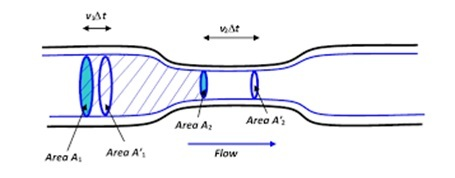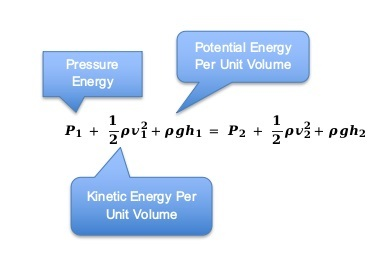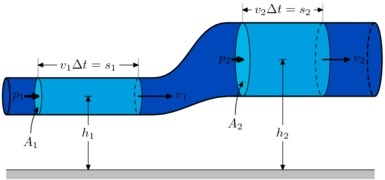# Bernoulli’s Principle

## Introduction

Bernoulli’s principle can be underst ood it with a simple explanation that when fluid goes from a high-diameter pipe to a low-diameter duct, the speed of the fluid increases many times. Yes, it is true, but is it that simple? Not at all. This principle is quite complex, with many formulas and factors controlling it. There are many applications worldwide utilizing this beautiful principle. One of the common examples explaining Bernoulli's principle is that the pull you feel towards the train in a railway station when a train passes through the platform, and if you are waiting near the platform side, is due to this principle. The moving train causes the pressure to be low near it. This low pressure pulls near objects towards it. Let us see in detail all about Bernoulli’s principle in this article.

## What is Bernoulli’s Principle?

The principle states that in a streamlined fluid flow, the sum of different energies along the line will be the same and constant. The three energies are kinetic energy, potential energy, and the external pressure applied to the fluid. Assume that the pipe has a raise from the ground level at one point, then that part of the pipe adds more potential energy due to the height difference. When the pipe diameter reduces in the streamline, the fluid velocity will increase, and as a result, the kinetic energy will increase.

## Bernoulli’s Principle Formula

The formula of this concept is more or less connected with the energy conservation law. The total energy of a streamlined fluid flow at any point in time and position in the pipeline is equal. As you refer to the figure below, the c enter portion of the pipe is narrow, and you can observe that the velocity of the fluid and hence the kinetic energy at that portion is more. So, there are such inferences here to discern.When the diameter of a pipe decrease, the cross-section area reduces, flow speed and pressure increase, and flow rate gets reduced.

$$\mathrm{P+\frac{1}{2}\rho v^2+\rho gh=constant}$$## Bernoulli’s Equation Derivation

The two criteria to be remembered while dealing with this equation is that the fluid should not be viscous and the fluid flow should be laminar. Referring to the below diagram, we will derive Bernoulli’s equation.anonymous, BernoullisLawDerivationDiagram, CC BY-SA 3.0

For the fluid flow to happen, we know there is an external work done, and this work when negotiated with other work like gravitational force results in a pressure difference all along the fluid path. So, work done is given by

$$\mathrm{W=F_1 dx_1-F_2 dx_2}$$

$\mathrm{dx_1=}$ length of a narrow tube

$\mathrm{dx_2=}$ length of a broad tube

Pressure is equivalent to force per unit area.

I.e. $\mathrm{P=\frac{F}{A}}$

So

$$\mathrm{F=P×A}$$

Substituting for force in above equation,

$$\mathrm{dW=P_1 A_1 dx_1-P_2 A_2 dx_2}$$

$$\mathrm{dW=P_1 dV-P_2 dV=(P_1-P_2 )dV }$$

$$\mathrm{dV=Adx}$$

The variation in kinetic energy is expressed as below.

$$\mathrm{dK=\frac{1}{2}m_1v_1^2-\frac{1}{2}m_2v_2^2}$$

Note, m is the mass and v is the velocity of a section of fluid.

Since, $\mathrm{mass = density\:\times \:volume}$

$$\mathrm{dK=\frac{1}{2}\rho dV(v_2^2-v_1^2)}$$

Now to find the change in potential energy,

$$\mathrm{ dU=mgh_2-mgh_1=ρdVg(h_2-h_1)\:\:\:\:dW=dK+dU}$$

$$\mathrm{(P_1-P_2)dV=\frac{1}{2}\rho dV(v_2^2-v_1^2)}$$

Cancelling dV on both sides, we get

$$\mathrm{(P_1-P_2)=\frac{1}{2}\rho (v_2^2-v_1^2 )+\rho g(h_2-h_1)}$$

On rearranging, above equation, we get Bernoulli's equation as given below.

$$\mathrm{P_1+\frac{1}{2}\rho v_1^2+\rho gh_1=P_2+ \frac{1}{2} \rho v_2^2+\rho gh_2}$$

## Principle of Continuity

The product of the cross-sectional area of the pipe and the velocity of the fluid at any point is always a constant. This is the principle of continuity. If $\mathrm{A_1}$ and $\mathrm{A_2}$ is the cross-sectional area of both the ends and $\mathrm{V_1}$ and $\mathrm{V_2}$ be the velocity of fluid at the respective ends, then we can write,

$$\mathrm{A_1 V_1 = A_2 V_2}$$

## Bernoulli’s Principle Use

The fundamentals of this principle are put together in the design elements of airplane aerodynamics, used in expert studies of fluid dynamics and experiments, and the design study of ferry boats where two boats are tied side by side, along with others.

## Relation Between Conservation of Energy and Bernoulli’s Equation

The equation states the principle of lowered pressure when the flow velocity increase in laminar fluid flows. So, for non-viscous fluids, the energy conservation is happening in a mutual way between the energy of motion, static energy, and pressure energy.

## Bernoulli’s Equation at Constant Depth

It is a unique way to consider the change in the equation when the “constant depth” scenario is conceived. In this scenario, we need to make both the heights h1 and h2 equal. That is h1=h2. Then the equation simplifies.

$$\mathrm{P_1+\frac{1}{2}\rho v_1^2=P_1+\frac{1}{2}\rho v_2^2}$$

## Bernoulli’s Equation for Static Fluids

The equation can be applied to static fluids, where the fluid is not moving. It is static. That means, we can give the value 0 to $\mathrm{v_1}$ and $\mathrm{v_2}$ in the equation. Then the equation reduces to

$$\mathrm{P_1+\rho gh_1=P_2+\rho gh_2}$$

## Bernoulli’s Principle Example

Whenever there is a deviation of an object either for a good purpose or as a natural reaction, and this happens due to the movement of air or fluids which are non-viscous, then there is Bernoulli’s principle embedded there.

• Airplanes lift due to high-pressure air generated from the propeller under the wings create a low pressure which lift the plane up.

• When a tornado leashes on top of a fixed roof, the roof flies away when the pressure becomes low due to the tornado's airspeed on top of the roof.

• A cricket ball swings due to the specific way the bowler throws the ball, and due to the reason, that bowler polishes one hemisphere brightly and keeps the other hemisphere of the ball rough.

• A fast train moving creates a pull due to its sheer speed which creates a low-pressure area in its path for some time. Chimney, atomizer, and so forth, you have a lot of everyday experiences of Bernoulli’s principle.

## Conclusion

Bernoulli’s principle applies to smooth flowing fluids. When a fluid flows through a pipe with two cross-sectional areas, a lesser diameter pipe creates more speed and pressure for fluid while the flow rate decrease. This phenomenon can be observed in various instances in day-to-day life. Since this principle takes lead from the energy conservation principle and defines an equation to describe the actions, this principle is applied in many critical application designs like the aerodynamics of the airplane.

## FAQs

Q1. How is the level flow of a fluid related to Bernoulli’s principle?

Ans. Level flow can be treated as constant depth. You have to take $\mathrm{h_1=h_2}$ in the equation.

Q2. What are entrainment devices?

Ans. Entrainment devices are devices where high-pressure fluids are made to flow using external force, which then entrain one fluid to another. Examples are atomizers, paint sprayers, and carburettors of vehicles.

Q3. What are the three heads applied in Bernoulli’s equation?

Q4. What is energy conservation law, from where Bernoulli’s principle is derived?

Ans. The energy conservation law states the principle of a constant sum of all energies acting on an object. When the potential energy (as height increases) of an object increases, its associated kinetic energy will decrease to make the total sum a constant. The equation of energy conservation law is

$$\mathrm{\frac{1}{2}mv^2+mgh=constant}$$

Q5. What is Venturi Effect?

Ans. It is a narrow tubing and due to low pressure thus created, is used to increase the velocity of fluids. Venturi Effect is another name to the principle we have been discussing all along.

Updated on: 28-Apr-2023

486 Views